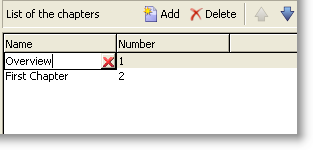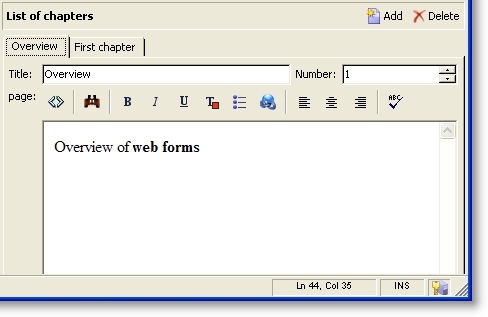v7 v8

# 輸入表單

Forms的詳細資訊，請參閱 本節.

## 表單結構

``````<form name="form_name" namespace="name_space">
…
</form>
````````````<form name="book" namespace="cus" type="contentForm">
<input xpath="@name"/>
<input xpath="@date"/>
<input xpath="@language"/>
</form>
``````

XPath是一種語法，可讓您在XML檔案的樹狀結構中找出節點。

• @date：選取名稱為「date」的屬性
• chapter/@title：選取「 」底下的「title」屬性 `<chapter>` 元素
• …/@date：從目前元素的父元素中選取日期

## 清單型別控制項

### 欄清單``````<input xpath="chapter" type="list">
<input xpath="@name"/>
<input xpath="@number"/>
</input>
````````````<input nolabel="true" toolbarCaption="List of chapters" type="list" xpath="chapter">
<input xpath="@name"/>
<input xpath="@number"/>
</input>
``````

toolbarCaption 屬性會強制工具列的水準對齊，並填入清單上方的標題。

#### 放大清單

• 為了方便資訊輸入，
• 存在多行控制項，
• 清單中的欄位僅包含主要欄位，而表單會顯示收集要素的所有欄位。``````<input nolabel="true" toolbarCaption="List of chapters" type="list" xpath="chapter" zoom="true" zoomOnAdd="true">
<input xpath="@name"/>
<input xpath="@number"/>

<form colcount="2" label="Editing a chapter">
<input xpath="@name"/>
<input xpath="@number"/>
<input colspan="2" xpath="page"/>
</form>
</input>
``````

A Detail 按鈕會在以下情況下自動新增： zoom="true" 在清單定義中輸入attribute。 這可讓您在選取的行上開啟編輯表單。

### 索引標籤清單``````<container toolbarCaption="List of chapters" type="notebooklist" xpath="chapter" xpath-label="@name">
<container colcount="2">
<input xpath="@name"/>
<input xpath="@number"/>
<input colspan="2" xpath="page"/>
</container>
</container>
``````

## 編輯表單Preview 索引標籤可讓您檢視輸入表單：